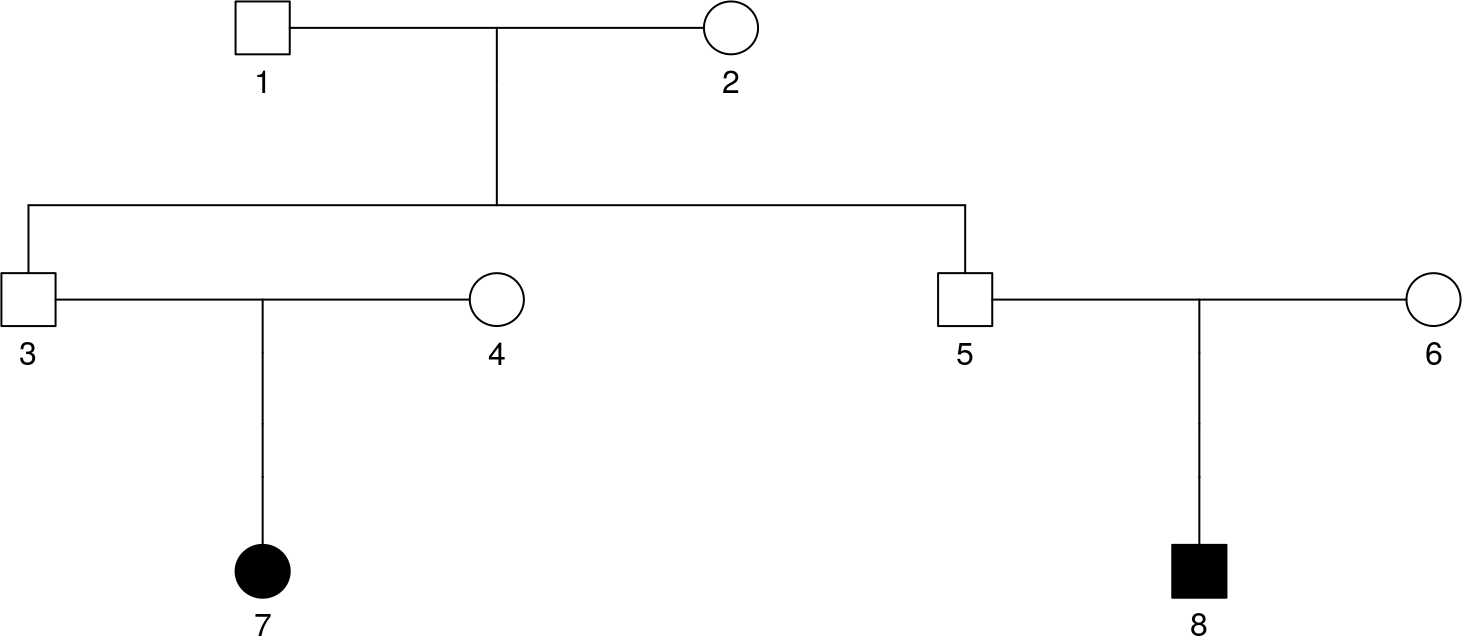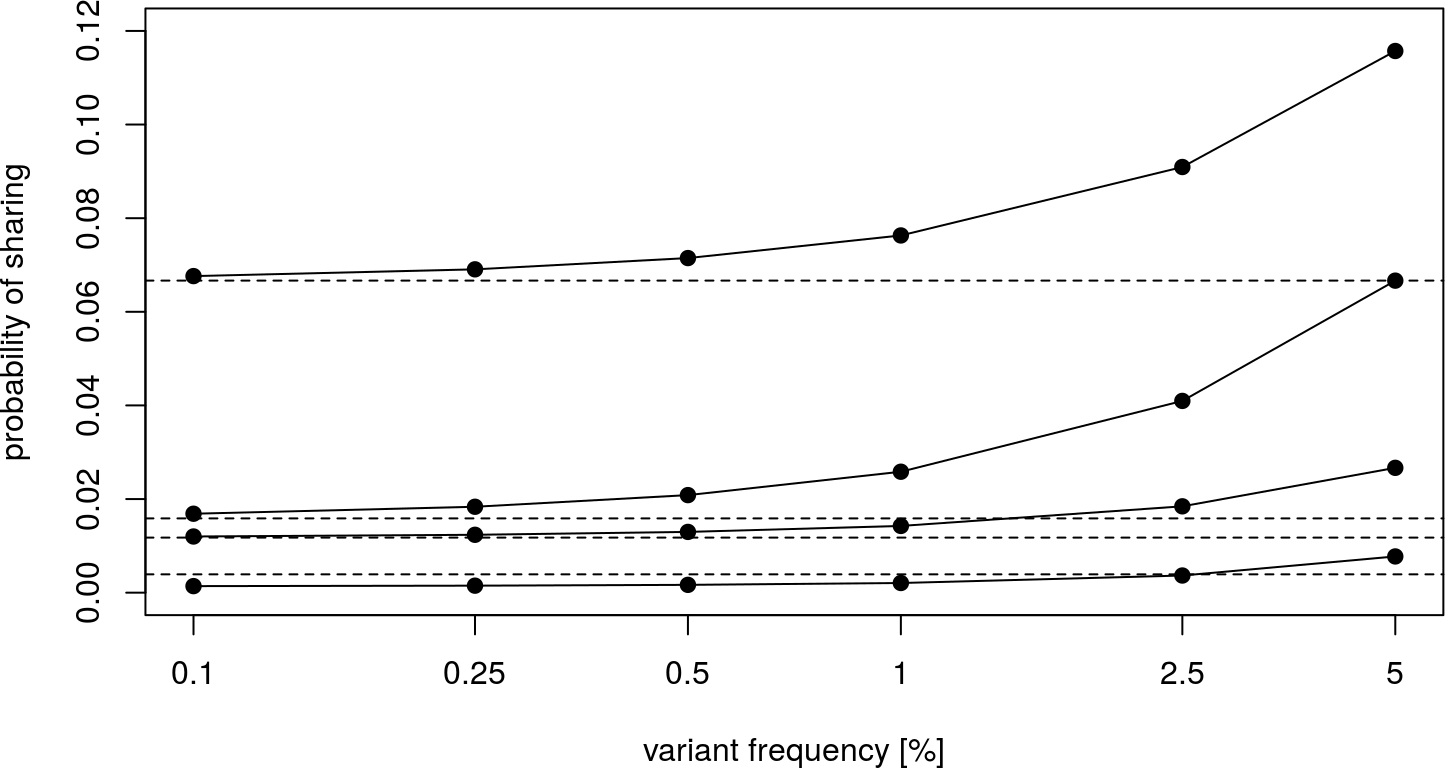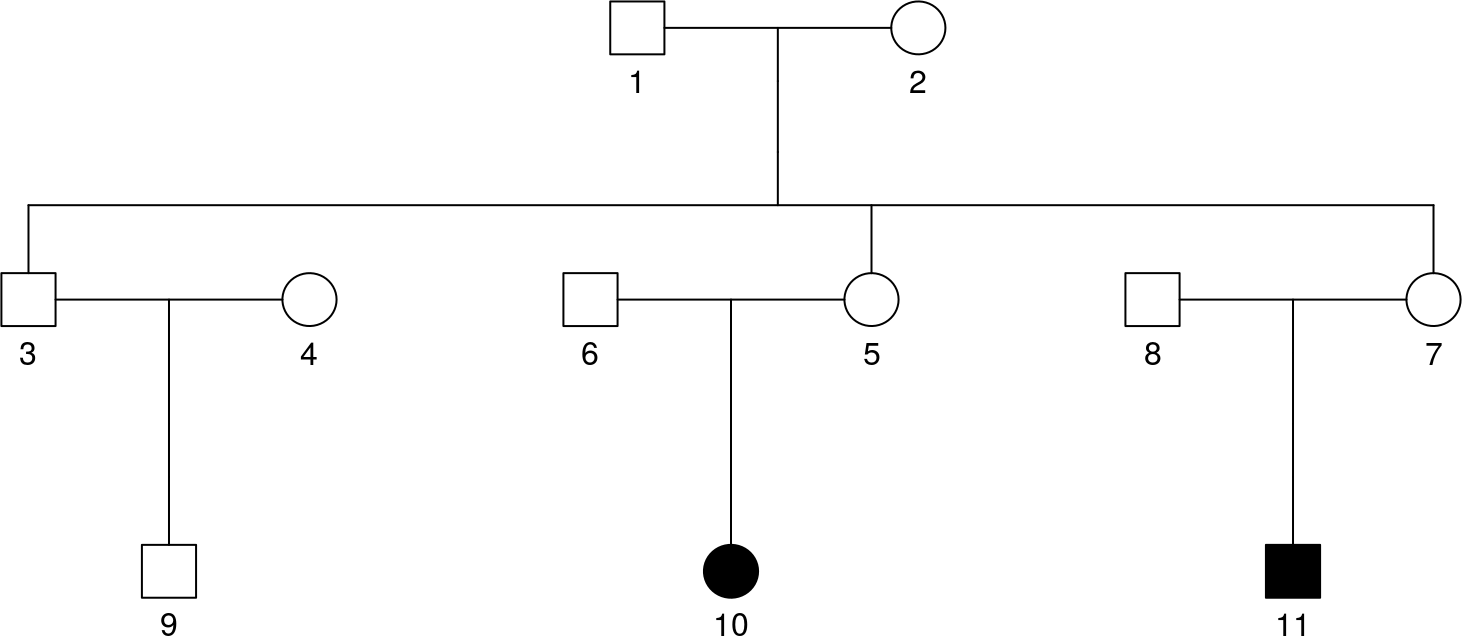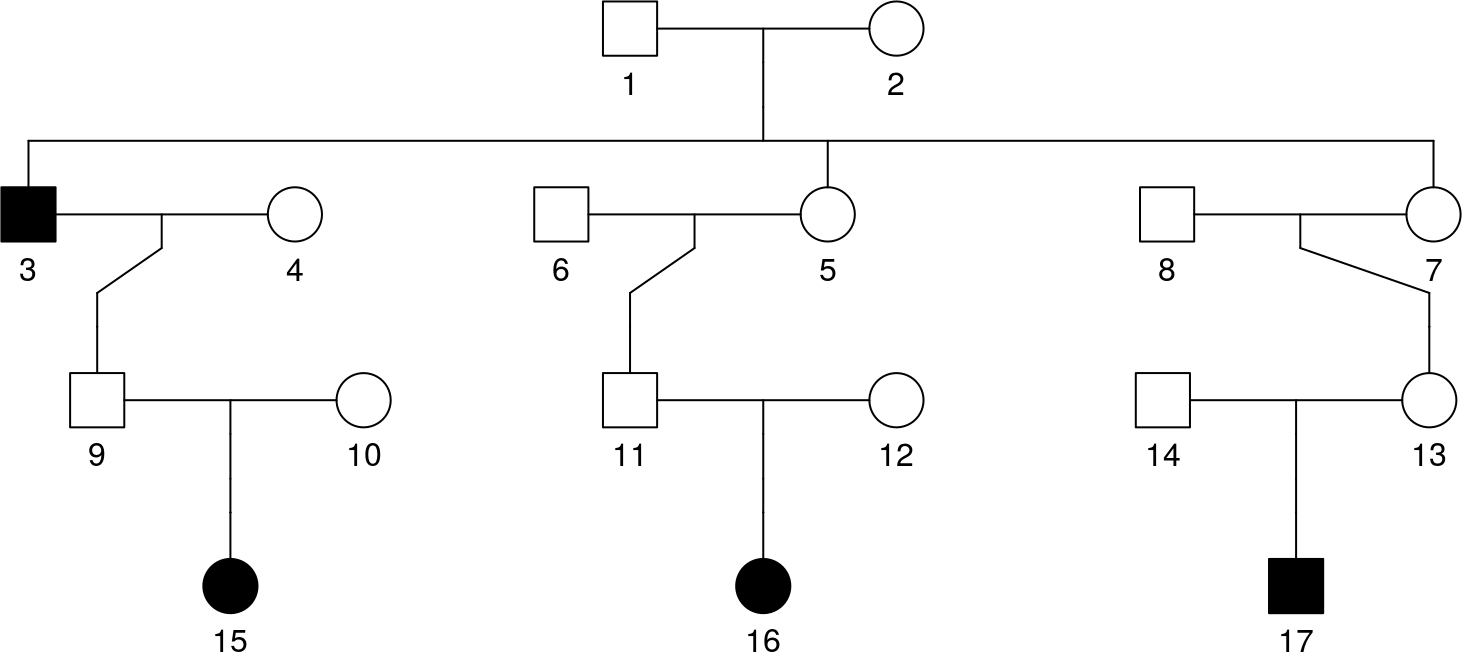# 1 Introduction

The primary use of the RVS package is to compute the rare variant (RV) sharing probabilities outlined in Bureau et al. (2014).

# 2 Pedigree format

The main input used in this package is a pedigree object from the kinship2 package on CRAN (Therneau et al. (2015)). The package vignette (https://cran.r-project.org/web/packages/kinship2/vignettes/pedigree.pdf) outlines the basic steps to creating a pedigree. Only the id, findex, mindex fields are necessary for computing sharing probabilities. The RVS package comes with 8 sample pedigrees.

data(samplePedigrees) # load sample pedigrees
kinship2::plot.pedigree(samplePedigrees$firstCousinPair) # plot pedigree# 3 Computing Standard Sharing Probabilities The primary function for computing sharing probabilities is RVsharing. There are two simple cases in which the calculation is straightforward. ## 3.1 Assuming One Founder Introduces the Variant In this case, we assume the variant is rare enough so that the probability of more than one founder introducing it to the pedigree is negligible. This is the default scenario for RVsharing. We define the following random variables: $$C_i$$: Number of copies of the RV received by subject $$i$$, $$F_j$$: Indicator variable that founder $$j$$ introduced one copy of the RV into the pedigree, For a set of $$n$$ subjects descendants of $$n_f$$ founders we want to compute the probability $\begin{eqnarray*} P[\mbox{RV shared}] &=& P[C_1 = \dots = C_n = 1 | C_1 + \dots + C_n \geq 1] \nonumber \\[0.5em] &=& \frac{P[C_1 = \dots = C_n = 1 ]}{P[C_1 + \dots + C_n \geq 1]} \nonumber \\[0.5em] &=& \frac{\sum_{j=1}^{n_f} P[C_1 = \dots = C_n = 1 | F_j] P[F_j]} {\sum_{j=1}^{n_f} P[C_1 + \dots + C_n \geq 1 | F_j]P[F_j]}, \label{sharingp} \end{eqnarray*}$ where the expression on the third line results from our assumption of a single copy of that RV among all alleles present in the $$n_f$$ founders. The probabilities $$P[F_j] = {1 \over n_f}$$ cancel from the numerator and denominator. RVsharing(samplePedigrees$firstCousinPair)
## Probability subjects 7 8 among 7 8 share a rare variant: 0.06667
##  0.06666667

## 3.2 When Allele Frequency is Known in the Founder Population

In this case, we know the allele frequency of the rare variant in the population the founders are drawn from. This allows for quick, exact calculation of the sharing probability. To specify the allele frequency, use the argument alleleFreq.

defaultProbs <- sapply(samplePedigrees[1:4], function(p)
suppressMessages(RVsharing(p)))

exactProbs <- list()
freq <- c(0.001,0.0025,0.005,0.01,0.025,0.05)
exactProbs$fistCousinPair <- sapply(freq, function(f) suppressMessages( RVsharing(samplePedigrees$firstCousinPair, alleleFreq=f)))
exactProbs$secondCousinPair <- sapply(freq, function(f) suppressMessages( RVsharing(samplePedigrees$secondCousinPair, alleleFreq=f)))
exactProbs$firstCousinTriple <- sapply(freq, function(f) suppressMessages( RVsharing(samplePedigrees$firstCousinTriple, alleleFreq=f)))
exactProbs$secondCousinTriple <- sapply(freq, function(f) suppressMessages( RVsharing(samplePedigrees$secondCousinTriple, alleleFreq=f)))

plot(NULL, xlim=c(0.001,0.05), ylim=c(0,0.12),log='x',xaxt='n',
ylab='probability of sharing', xlab='variant frequency [%]')
axis(side=1, at=freq, labels=freq*100)
invisible(sapply(exactProbs, lines, x=freq))
invisible(sapply(exactProbs, points, x=freq, pch=19))
abline(h=defaultProbs,lty=2)Similiar to Figure 2 in Bureau et al. (2014)

## 3.3 Sharing Probabilties in a Subset of a Pedigree

By default, RVsharing will compute the probability that all of the final descendants share the variant given that it is seen in at least one of them. Final descendants are defined as subjects of the pedigree with no children. This event can be customized with the carriers and useAffected arguments.

If the argument carriers is provided, then the probability of all carriers having the variant given it is seen in at least one final descendant will be computed.

If the argument useAffected is TRUE and the pedigree has a slot for affected, then the probability of all carriers having the variant given it is seen in at least one affected will be computed.

These two arguments can be used individually or in combination, the only restriction is that carriers must be a subset of affected.

ped <- samplePedigrees$firstCousinTriple ped$affected <- 0
plot(ped)p <- RVsharing(ped)
## Probability subjects 9 10 11 among 9 10 11 share a rare variant: 0.01176
p <- RVsharing(ped, useAffected=TRUE)
## Probability subjects 10 11 among 10 11 share a rare variant: 0.06667
p <- RVsharing(ped, carriers=c(9,10))
## Probability subjects 9 10 among 9 10 11 share a rare variant: 0.03529
p <- RVsharing(ped, carriers=c(10,11), useAffected=TRUE)
## Probability subjects 10 11 among 10 11 share a rare variant: 0.06667

# 5 Using Monte Carlo Simulation

RVsharing also allows for estimating sharing probabilities through monte carlo simulation. The primary use of this feature is for calculating sharing probabilities under non standard assumptions about the founders. However, this feature is available for the standard assumptions as well. To run a monte carlo simulation, specify all parameters as normal and additionally provide the nSim parameter specifying how many simulations should be run.

## 5.1 Standard Sharing Probabilties

p <- RVsharing(samplePedigrees$firstCousinPair) ## Probability subjects 7 8 among 7 8 share a rare variant: 0.06667 p <- RVsharing(samplePedigrees$firstCousinPair, nSim=1e4)
## Probability subjects 7 8 among 7 8 share a rare variant: 0.07032
p <- RVsharing(samplePedigrees$firstCousinPair, alleleFreq=0.01) ## Probability subjects 7 8 among 7 8 share a rare variant: 0.07631 p <- RVsharing(samplePedigrees$firstCousinPair, alleleFreq=0.01, nSim=1e4)
## Probability subjects 7 8 among 7 8 share a rare variant: 0.07494
p <- RVsharing(samplePedigrees$firstCousinPair, kinshipCoeff=0.05) ## Probability subjects 7 8 among 7 8 share a rare variant: 0.1262 p <- RVsharing(samplePedigrees$firstCousinPair, kinshipCoeff=0.05, nSim=1e4)
## Probability subjects 7 8 among 7 8 share a rare variant: 0.1195

## 5.2 Custom Founder Distributions

This method allows for more complex relationships among the founders to be given. RVsharing allows for a complete distribution among the founders to be passed in as the parameter founderDist. This function should accept a single argument, N, and should return a vector of length N with values in {0,1,2} representing the number of copies of the variant each founder has.

# assumption that 1 founder introduces
fDist <- function(N) sample(c(rep(0,N-1), 1))
RVsharing(samplePedigrees$firstCousinPair, nSim=1e4, founderDist=fDist) ## Probability subjects 7 8 among 7 8 share a rare variant: 0.05992 ##  0.05991868 RVsharing(samplePedigrees$firstCousinPair)
## Probability subjects 7 8 among 7 8 share a rare variant: 0.06667
##  0.06666667

# 6 Calculating Sharing Probabilties Across Multiple Families

For variants seen in only one family, the sharing probability can be interpreted directly as a P-value from a Bernoulli trial. For variants seen in M families and shared by affected relatives in a subset S of them, the P-value can be obtained as the sum of the probability of events as (or more) extreme as the observed sharing in the family subset S. The function multipleFamilyPValue takes a vector of sharing probabilities and a vector of TRUE/FALSE describing whether or not the variant was shared among the carriers.

probs <- sapply(samplePedigrees, function(p) suppressMessages(RVsharing(p)))
observed <- c(rep(FALSE, 3), rep(TRUE, 2), rep(FALSE, 4))
multipleFamilyPValue(probs, observed)
##  0.0002114833

# 7 Precomputing Sharing Probabilities and Number of Carriers for all Possible Carrier Subsets

The RVgene function requires the lists pattern.prob.list of vectors of sharing probabilities and N.list of number of carriers for all possible affected carrier subsets in each family in the sample being analyzed. The elements of both of these lists must have the same names as the pedigree objects in the ped.listfams argument. When all affected subjecs in a family are final descendants, the sharing probabilities and number of carriers for all subsets can be generated automatically. Here is an exanple with three second cousins:

carriers = c(15,16,17)
carrier.sets = list()
for (i in length(carriers):1)
carrier.sets = c(carrier.sets, combn(carriers,i,simplify=FALSE))
fam15157.pattern.prob = sapply(carrier.sets,function (vec) RVsharing(samplePedigrees$secondCousinTriple,carriers=vec)) ## Probability subjects 15 16 17 among 15 16 17 share a rare variant: 0.001342 ## Probability subjects 15 16 among 15 16 17 share a rare variant: 0.009396 ## Probability subjects 15 17 among 15 16 17 share a rare variant: 0.009396 ## Probability subjects 16 17 among 15 16 17 share a rare variant: 0.009396 ## Probability subjects 15 among 15 16 17 share a rare variant: 0.3235 ## Probability subjects 16 among 15 16 17 share a rare variant: 0.3235 ## Probability subjects 17 among 15 16 17 share a rare variant: 0.3235 fam15157.N = sapply(carrier.sets,length) While this code applies to any configuration of affected final descendants, symmetries in the relationships of these third cousins results in equal sharing probabilities for multiple subsets. Subsets with the same probabilities are equivalent, and the optional argument nequiv.list can be used to indicate the number of equivalent subset for each sharing probability. While shorter vectors in pattern.prob.list and N.list result in more efficient computation, identification of the equivalent subsets is not easily automated, and will usually require custom code for each pedigree in a sample. With three second cousins we can use: fam15157.pattern.prob = c(RVsharing(samplePedigrees$secondCousinTriple,carriers=c(15,16,17)),
RVsharing(samplePedigrees$secondCousinTriple,carriers=c(15,16)), RVsharing(samplePedigrees$secondCousinTriple,carriers=c(15)))
## Probability subjects 15 16 17 among 15 16 17 share a rare variant: 0.001342
## Probability subjects 15 16 among 15 16 17 share a rare variant: 0.009396
## Probability subjects 15 among 15 16 17 share a rare variant: 0.3235
fam15157.N = 3:1
fam15157.nequiv = c(1,3,3)

It is then easy to check that the distribution sums to 1:

sum(fam15157.pattern.prob*fam15157.nequiv)
##  1

When some affected subjects are not final descendants, some subsets are incompatible with a variant being IBD among carriers. Assume individual 3, the grand-father of subject 15 in family 15157, is also affected and his genotype is available.

fam15157 <- samplePedigrees$secondCousinTriple fam15157$affected = 1
plot(fam15157)Then the carrier subsets (15,16,17), (15,16) and (15,17) involving subject 15 but not 3 are incompatible with sharing IBD and must be removed from the list of subsets. The code then becomes:

carriers = c(3,15,16,17)
carrier.sets = list()
for (i in length(carriers):1)
carrier.sets = c(carrier.sets, combn(carriers,i,simplify=FALSE))
carrier.sets
## []
##   3 15 16 17
##
## []
##   3 15 16
##
## []
##   3 15 17
##
## []
##   3 16 17
##
## []
##  15 16 17
##
## []
##   3 15
##
## []
##   3 16
##
## []
##   3 17
##
## []
##  15 16
##
## []
##  15 17
##
## []
##  16 17
##
## []
##  3
##
## []
##  15
##
## []
##  16
##
## []
##  17
carrier.sets = carrier.sets[-c(5,9,10)]
fam15157.pattern.prob = sapply(carrier.sets,function (vec) RVsharing(fam15157,carriers=vec,useAffected=TRUE))
## Probability subjects 3 15 16 17 among 3 15 16 17 share a rare variant: 0.001121
## Probability subjects 3 15 16 among 3 15 16 17 share a rare variant: 0.007848
## Probability subjects 3 15 17 among 3 15 16 17 share a rare variant: 0.007848
## Probability subjects 3 16 17 among 3 15 16 17 share a rare variant: 0.003363
## Probability subjects 3 15 among 3 15 16 17 share a rare variant: 0.05493
## Probability subjects 3 16 among 3 15 16 17 share a rare variant: 0.02354
## Probability subjects 3 17 among 3 15 16 17 share a rare variant: 0.02354
## Probability subjects 16 17 among 3 15 16 17 share a rare variant: 0.004484
## Probability subjects 3 among 3 15 16 17 share a rare variant: 0.1648
## Probability subjects 15 among 3 15 16 17 share a rare variant: 0.2152
## Probability subjects 16 among 3 15 16 17 share a rare variant: 0.2466
## Probability subjects 17 among 3 15 16 17 share a rare variant: 0.2466
fam15157.N = sapply(carrier.sets,length)

Notice the use of the option useAffected=TRUE with affected subjects who are not final descendants. Again, one can check that the distribution sums to 1:

sum(fam15157.pattern.prob)
##  1

Precomputed sharing probabilities and numbers of carriers can be used directly to obtain p-values of observed sharing events, by summing the probability of all events as or more extreme as the one observed (both in terms of sharing probability and number of carriers), i.e. this is a one-sided exact test. For instance, if subjects 3, 16 and 17 share a rare variant, the probability of that event is

pobs = RVsharing(fam15157,carriers=c(3,16,17),useAffected=TRUE)
## Probability subjects 3 16 17 among 3 15 16 17 share a rare variant: 0.003363

The p-value of that sharing event is then:

sum(fam15157.pattern.prob[fam15157.pattern.prob<=pobs & fam15157.N >= 3])
##  0.004484305

The RVgene function enables these computations with more than one family harboring the same or different variants. Once the vectors of sharing probabilities and number of carriers have been computed for all families in the sample, they need to be stored in lists. We return to the original second cousin triple family and add a first and second cousin triple family. Then we create the lists of pattern probabilities, number of equivalent subsets and number of carriers in the subsets.

fam15157.pattern.prob = c(RVsharing(samplePedigrees$secondCousinTriple,carriers=c(15,16,17)), RVsharing(samplePedigrees$secondCousinTriple,carriers=c(15,16)),
RVsharing(samplePedigrees$secondCousinTriple,carriers=c(15))) ## Probability subjects 15 16 17 among 15 16 17 share a rare variant: 0.001342 ## Probability subjects 15 16 among 15 16 17 share a rare variant: 0.009396 ## Probability subjects 15 among 15 16 17 share a rare variant: 0.3235 fam15157.N = 3:1 fam15157.nequiv = c(1,3,3) fam28003.pattern.prob = c(RVsharing(samplePedigrees$firstAndSecondCousinsTriple,carriers=c(36,104,110)),
RVsharing(samplePedigrees$firstAndSecondCousinsTriple,carriers=c(36,104)), RVsharing(samplePedigrees$firstAndSecondCousinsTriple,carriers=c(104,110)),
RVsharing(samplePedigrees$firstAndSecondCousinsTriple,carriers=c(36)), RVsharing(samplePedigrees$firstAndSecondCousinsTriple,carriers=c(104)))
## Probability subjects 36 104 110 among 36 104 110 share a rare variant: 0.00277
## Probability subjects 36 104 among 36 104 110 share a rare variant: 0.00831
## Probability subjects 104 110 among 36 104 110 share a rare variant: 0.04155
## Probability subjects 36 among 36 104 110 share a rare variant: 0.3352
## Probability subjects 104 among 36 104 110 share a rare variant: 0.3019
fam28003.N = c(3,2,2,1,1)
fam28003.nequiv = c(1,2,1,1,2)

ex.pattern.prob.list = list("15157"=fam15157.pattern.prob,"28003"=fam28003.pattern.prob)
ex.nequiv.list = list("15157"=fam15157.nequiv,"28003"=fam28003.nequiv)
ex.N.list = list("15157"=fam15157.N,"28003"=fam28003.N)

# 8 Analyzing variants in genomic sequence

We now turn to the analysis of variants observed in the simulated genomic sequence of the gene PEAR1 in a sample of related affected subjects. The processing of the sequence data results in Variant Call Format (VCF) files, which can be read into R with the function readVcf from the variantAnnotatin package. Two VCF objects obtained with readVcf from VCF files of sequence data for the second cousin triple and first and second cousin triple families are contained in the famVCF data. These VCF files are converted to snpMatrix objects using the genotypeToSnpMatrix function.

data(famVCF)
library(VariantAnnotation)
## Loading required package: BiocGenerics
## Loading required package: parallel
##
## Attaching package: 'BiocGenerics'
## The following objects are masked from 'package:parallel':
##
##     clusterApply, clusterApplyLB, clusterCall, clusterEvalQ,
##     clusterExport, clusterMap, parApply, parCapply, parLapply,
##     parLapplyLB, parRapply, parSapply, parSapplyLB
## The following objects are masked from 'package:Matrix':
##
##     colMeans, colSums, rowMeans, rowSums, which
## The following objects are masked from 'package:stats':
##
##     IQR, mad, sd, var, xtabs
## The following objects are masked from 'package:base':
##
##     Filter, Find, Map, Position, Reduce, anyDuplicated, append,
##     as.data.frame, cbind, colMeans, colSums, colnames, do.call,
##     duplicated, eval, evalq, get, grep, grepl, intersect,
##     is.unsorted, lapply, lengths, mapply, match, mget, order, paste,
##     pmax, pmax.int, pmin, pmin.int, rank, rbind, rowMeans, rowSums,
##     rownames, sapply, setdiff, sort, table, tapply, union, unique,
##     unsplit, which, which.max, which.min
## Loading required package: GenomeInfoDb
## Loading required package: S4Vectors
## Loading required package: stats4
##
## Attaching package: 'S4Vectors'
## The following object is masked from 'package:Matrix':
##
##     expand
## The following object is masked from 'package:base':
##
##     expand.grid
## Loading required package: IRanges
## Loading required package: GenomicRanges
## Loading required package: SummarizedExperiment
## Loading required package: Biobase
## Welcome to Bioconductor
##
##     Vignettes contain introductory material; view with
##     'browseVignettes()'. To cite Bioconductor, see
##     'citation("Biobase")', and for packages 'citation("pkgname")'.
## Loading required package: DelayedArray
## Loading required package: matrixStats
##
## Attaching package: 'matrixStats'
## The following objects are masked from 'package:Biobase':
##
##     anyMissing, rowMedians
##
## Attaching package: 'DelayedArray'
## The following objects are masked from 'package:matrixStats':
##
##     colMaxs, colMins, colRanges, rowMaxs, rowMins, rowRanges
## The following object is masked from 'package:base':
##
##     apply
## Loading required package: Rsamtools
## Loading required package: Biostrings
## Loading required package: XVector
##
## Attaching package: 'Biostrings'
## The following object is masked from 'package:DelayedArray':
##
##     type
## The following object is masked from 'package:base':
##
##     strsplit
##
## Attaching package: 'VariantAnnotation'
## The following object is masked from 'package:base':
##
##     tabulate
fam15157.snp = genotypeToSnpMatrix(fam15157.vcf)
## non-single nucleotide variations are set to NA
## Warning in .local(x, ...): non-diploid variants are set to NA
fam28003.snp = genotypeToSnpMatrix(fam28003.vcf)
## non-single nucleotide variations are set to NA
## Warning in .local(x, ...): non-diploid variants are set to NA

RVgene requires lists of the snpMatrix and pedigree objects for these two families. The names given to the elements of these lists are not used by RVgene and are thus arbitrary. Family IDs are extracted from the famid element of the pedigree objects. Please note that currently RVgene does not accept a pedigreeList, but only a plain list of pedigree objects.

ex.SnpMatrix.list = list(fam15157=fam15157.snp$genotypes,fam28003=fam28003.snp$genotypes)
ex.ped.obj = list(fam15157=samplePedigrees$secondCousinTriple,fam28003=samplePedigrees$firstAndSecondCousinsTriple)

In the sequence segment, one can specify which variants are rare and possibly satisfy other filtering criteria (e.g. coding variants) using the sites argument. Here, we will focus on two sites: 92 where the three second cousins of family 15157 share the rare allele and 119 where the two first cousins of family 28003 share the rare allele but not their second cousin.

sites = c(92,119)
ex.SnpMatrix.list[["fam15157"]][,sites]@.Data
##    rs187756653
## 1           00
## 2           00
## 3           00
## 4           00
## 5           00
## 6           00
## 7           00
## 8           00
## 9           00
## 10          00
## 11          00
## 12          00
## 13          00
## 14          00
## 15          02
## 16          02
## 17          02
ex.SnpMatrix.list[["fam28003"]][,sites]@.Data
##     rs199628333
## 3            00
## 4            00
## 6            00
## 7            00
## 11           00
## 12           00
## 13           00
## 15           00
## 27           00
## 28           00
## 36           01
## 103          00
## 104          02
## 109          00
## 110          02

Finally, the call to RVgene returns the P-value of the exact rare variant sharing test allowing for sharing by a subset of affected subjects (p), the P-value of the exact rare variant sharing test requiring sharing by all affected subjects (pall) and the minimum achievable p-value if all affected subjects were carriers of a rare variant (potentialp).

RVgene(ex.SnpMatrix.list,ex.ped.obj,sites,pattern.prob.list=ex.pattern.prob.list,
nequiv.list=ex.nequiv.list,N.list=ex.N.list,type="count")
## $p ##  0.000159884 ## ##$pall
##  0.001342282
##
## \$potentialp
##  3.718232e-06